# Insertion Loss

Insertion loss is almost universally expressed in decibels (dB), and is generally supposed to be a positive number but we forgive you in advance if you ever say "this attenuator has -9.54 dB insertion loss". Insertion loss is only defined for two port networks, but you could bastardize the definition to include the forward loss of a circulator, even if you are terminating one port to use as an isolator. Insertion loss and gain is what is used in cascade analysis.

We refer to Peter Rizzi's excellent book Microwave Engineering: Passive Circuits, whenever we are looking for a correct definition of anything to do with loss or gain. The explanation below was adapted from his work. Go to our book page and pay tribute by buying it!

Rizzi also points out the difference between the words attenuation, and loss, which we have mixed up on Microwaves101 numerous times. If you are talking about a transmission line, use the word attenuation when discussing how much power is lost per length. When discussing two-port circuits (such as attenuators), the correct term is loss.

## Insertion loss (or gain)

In order to understand insertion loss, study the two figures below. In figure (a) a generator with impedance ZG is hooked up to a load ZL. They are not necessarily matched.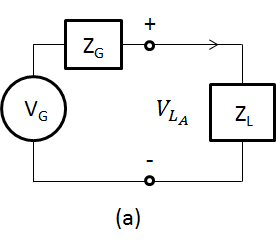In figure (b), a two-port network was inserted between them.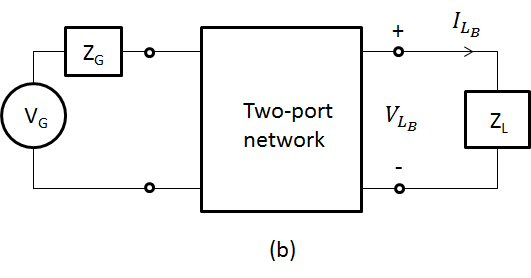If the two-port network is passive, we refer to insertion loss as: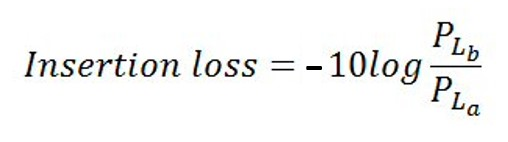(in decibels)

where PLa is the power delivered in figure (a) and PLb is the power delivered in figure (b).

Note that a passive network is not necessarily reciprocal (it could be a ferrite isolator for example).

Insertion loss what you are familiar with measuring when you are doing power meter measurements. Any mismatch loss is rolled up in the calculation. Note that S-parameters S21 and S12 (equal for a passive network) are often confused with insertion loss. They would be equal to insertion loss, if the load, generator and two-port network are all matched to the same Z0, which never happens in real life. For all intents and purposes, if your generator is well matched lab equipment (or matched using an isolator you added), and your are using good power head that is -20 dB matched to 50 ohms, and your network is -20 dB matched on both ports, then you could get away with this misusing S-parameters as insertion loss with immeasurable power error of 1% or less.

Note that if you observe loss in an insertion loss measurement, the quantity in dB is positive. But it is possible to have negative insertion loss (more power delivered to load in Figure (b)), more on that in a moment...

If the network is active (say, an amplifier), you should reverse the numerator and denominator to express insertion gain. This is what many engineers define insertion loss as, and express loss as a negative-dB quantity. No big deal, just roll your eyes and move on.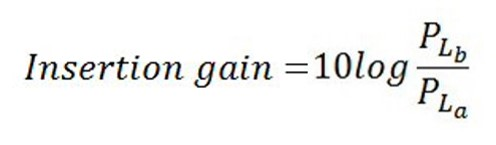(in decibels)

The definition of insertion loss allows a passive network to have negative loss. Think about a matching network: if you had a transistor connected to 50 ohms, then inserted a matching network, the power delivered to the load goes up if you did it right. Unless you are an amplifier designer, this probably will never come up. But if you are...

## Transducer loss (or gain)

Transducer loss eliminates the "problem" of negative insertion loss, where a passive network might increase delivered power.

Transducer loss is the decibel ratio of power available to power delivered to the load.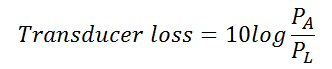(in decibels)

Similarly, transducer gain is: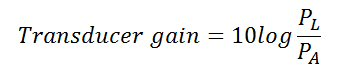(in decibels)

Look for other gain formulas on our gain page.

Author : Unknown Editor